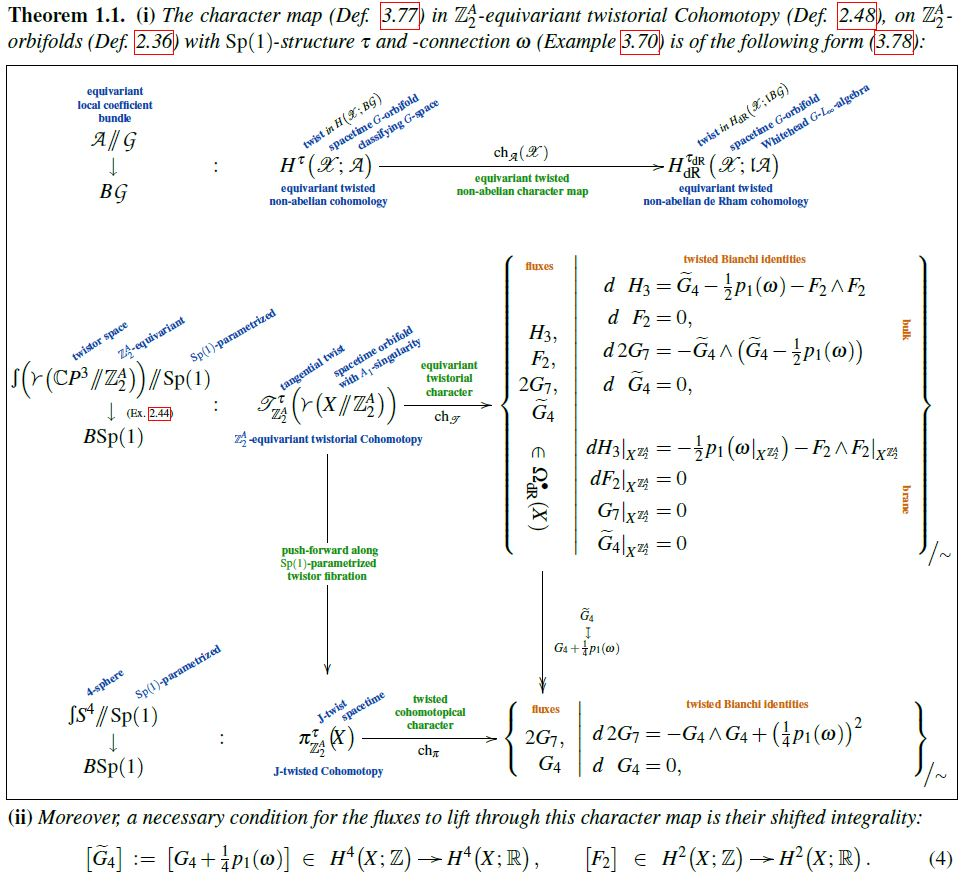Schreiber The Character Map in Equivariant Twistorial Cohomotopy

An article that we have written:

• The character map in equivariant twistorial Cohomotopy

implies the Green-Schwarz mechanism with heterotic M5-branes

Abstract. The celebrated Green-Schwarz mechanism in heterotic string theory has been suggested to secretly be a higher gauge theoretic phenomenon, embodying a higher Bianchi identity for a higher-degree analog of a curvature form of a higher gauge field.

Here we prove that the non-perturbative Hořava-Witten Green-Schwarz mechanism for heterotic line bundles in heterotic M-theory with M5-branes parallel to MO9-planes on A1-singularities is accurately encoded in the higher gauge theory for higher gauge group of the equivariant homotopy type of the $\mathbb{Z}_2$-equivariant $A_\infty$-loop group of twistor space. In this formulation, the flux forms of the heterotic gauge field, the B-field on the M5-brane and of the C-field in the M-theory bulk are all unified into the character image of a single cocycle in equivariant twistorial Cohomotopy theory; and that cocycle enforces the quantization condition on all fluxes: the integrality of the gauge flux, the half-shifted integrality of the C-field flux and the integrality of the dual C-field flux (i.e. of the Page charge in the bulk and of the Hopf-WZ term on the M5-brane). This result is in line with the Hypothesis H that M-brane charges are quantized in J-twisted Cohomotopy theory.

The mathematical heart of our proof is the construction of the equivariant twisted non-abelian character map via an equivariant twisted non-abelian de Rham theorem, which we prove; as well as the computation of the equivariant relative minimal model of the $\mathbb{Z}_2$-equivariant Sp(1)-parametrized twistor fibration. We lay out the relevant background in equivariant rational homotopy theory and explain how this brings about the subtle flux quantization relations in heterotic M-theory.Followup to

Related articles on Hypothesis H:

Last revised on December 23, 2021 at 08:09:13. See the history of this page for a list of all contributions to it.